## Canvas - Transforms

The Canvas API has 3 basic transform functions. What’s a little non-intuitive about these functions is they act on the canvas itself, and only indirectly on the canvas contents.

In Canvas - The Beginning the coordinate system was introduced with 0,0 defaulting to the top left corner of the canvas; which was kind of weird because the 2D plots most are first introduced to depict 0,0 in the center of the graph.

### Translate

The Translate function moves this 0,0 origin to an offset passed in the x,y argument. So to move the origin to the center of the canvas on the screen:

``ctx.translate(canvas.width / 2, canvas.height / 2);``

Transform functions effect what is drawn after the function, it doesn’t effect the contents that already on canvas. A rectangle drawn after the translate function call above:

``ctx.fillRect(0, 0, 25, 25);``

Results in this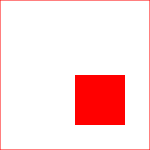Rather than this (if drawn before the translate):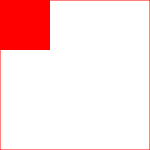Note that the rectangle is drawn from its top left corner. So to center the rectangle in the canvas after the translate, its x and y need to be adjusted:

``````var size = 50;
ctx.fillRect(-0.5*size,-0.5*size,size,size);
``````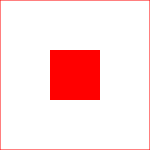### Rotate

Rotates a canvas around its current origin. That origin part is key to some nifty effects (and confusion sometimes.)

To rotate the rectangle drawn previously 45 degrees:

``ctx.translate(canvas.width / 2, canvas.height / 2);ctx.rotate((45 * 180) / Math.PI);var size = 50;ctx.fillRect(-0.5 * size, -0.5 * size, size, size);``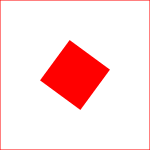the rotate function takes radians as its argument and the formulas for switching between degrees and radians are:

radians = degrees _ Math.PI / 180 degrees = radians _ 180 / Math.PI

Note this will rotate everything that is drawn after rotate is called until the transformations are reset back to default either through:

ctx.save() and ctx.restore() which saves and restores the state of the canvas, including the state of the transformations, fills, line sizes, etc (but not the graphics on the canvas.)

or

``ctx.setTransform(1, 0, 0, 1, 0, 0);``

which is faster, but just restores the state of the transformations back to their default settings.

### Scale

ctx.scale(x,y) scales pixels (by default) x horizontally and y vertically where x and y are real numbers and 1.0 = current size and < 1.0 shrinks and > 1.0 expands. So 0.5 pixels would be half size and 2.0 would be twice as large.

Negative values can create some interesting effects:

``// mirror horizontallyctx.scale(-1, 1);``

### Trig and the Circle

Trigonometry is all about the triangle. What makes it useful for navigating points and angles around a circle is illustrated in this diagram.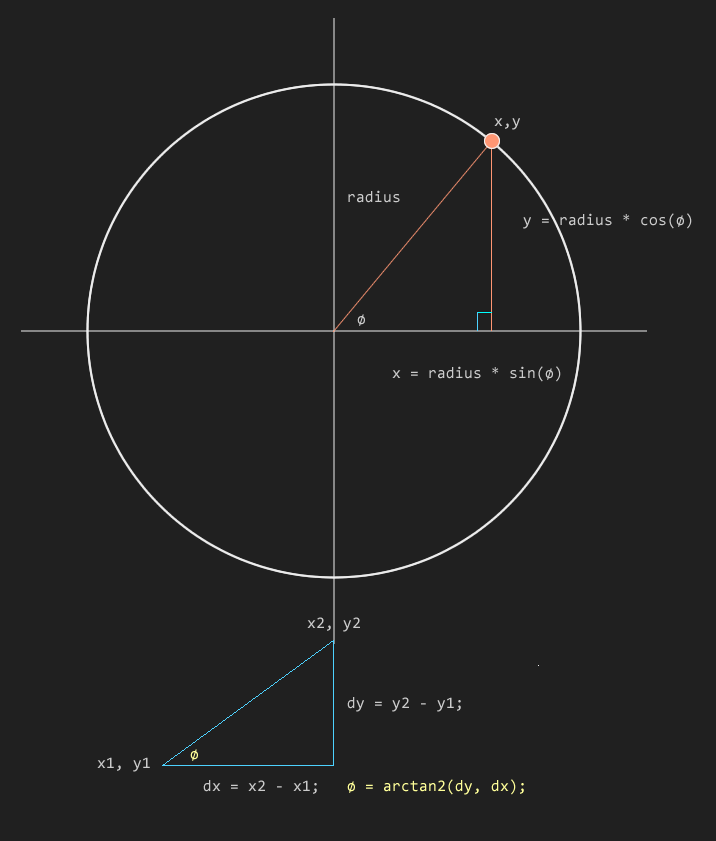Trig functions, like sine and cosine are ratios of different sides of triangles at different angles (in radians) and this ratio can be used to draw an object at a specific point on the perimeter of a circle of any radius.

Another trig function, the tangent, or rather an inverse variant of it Math.arctan2(), when given the length of two sides returns the angle. Very handy for rotating one thing to face another or reading a value off interface elements like dials.

Using a couple of these transforms, and some trigonometry, the next experiment explores aesthetic effects of rotation on a simple construct. Try playing with the rotation speed and blur sliders to see a range of visuals that can be created from a few spokes of rectangles.

See the Pen Triangle Spirals by Kentskyo (@kentskyo) on CodePen.